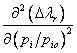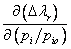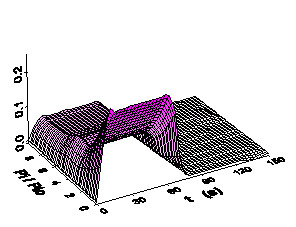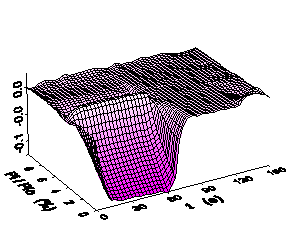Home News About Me Ph. D. Thesis Abstract Table of Contents 1. Introduction 2. Theory  Fundamentals of the Multivariate Data Analysis 3. Theory  Quantification of the Refrigerants R22 and R134a: Part I 4. Experiments, Setups and Data Sets 5. Results  Kinetic Measurements 6. Results  Multivariate Calibrations 6.1. PLS Calibration 6.1.1. Wald-Wolfowitz Runs Test 6.1.2. Durbin-Watson Statistics 6.1.3. Results of Statistical Tests 6.2. Box-Cox Transformation + PLS 6.3. INLR 6.4. QPLS 6.5. CART 6.6. Model Trees 6.7. MARS 6.8. Neural Networks 6.9. PCA-NN 6.10. Neural Networks and Pruning 6.11. Conclusions 7. Results  Genetic Algorithm Framework 8. Results  Growing Neural Network Framework 9. Results  All Data Sets 10. Results  Various Aspects of the Frameworks and Measurements 11. Summary and Outlook 12. References 13. Acknowledgements Publications Research Tutorials Downloads and Links Contact Search Site Map Print this Page### 6.1.3.   Results of Statistical Tests

For the residuals of the predictions of both analytes by all PLS models (Martens' Uncertainty Test and minimum crossvalidation criterion), the Wald-Wolfowitz Runs test (p=0.000) shows that the sequence of the residuals is highly non-random. The null hypotheses of the Durbin-Watson Statistics have to be rejected at the 5% error level for both analytes and both models indicating a correlation of the residuals. The combination of both statistics and the true-predicted plots indicate that the PLS cannot deal with nonlinearities, which are present in the relationship between the concentrations of the analytes and the signals of the device.

The nonlinearities, which are somehow visible in figure 22, can be made more prominent by plotting the first and second partial derivatives of the signals with respect to the relative saturation pressure versus the relative saturation pressure of the analyte R22 and versus time in figure 33. It is obvious that during exposure to R22 the first partial derivative is not constant along the concentration axis (y-axis) confirming that the relationship between the sensor signals and the relative saturation pressures of R22 is not linear. The partial derivative of the second order is neither zero nor constant. This means that the relationship also cannot be exactly described by polynomials with quadratic terms. The same nonlinear relationship between the signal and the relative saturation pressure can also be observed for R134a, but is not shown here. The nonlinearity can be ascribed to a saturation effect, as the number of micropores is limited (see discussion in section 3.2). For the high-concentrated mixtures, the nonlinearity is even worse since the total concentrations add up to 0.2 pi/pio. As for both analytes this nonlinearity can be observed and as a model for the nonlinear relationship between the concentrations and the signals is not known, several extensions to the linear models and several variable transformations are used for the calibration of this data set in the next sections. Additionally, "true nonlinear" methods, the neural networks, are applied to the data set in the last sections of this chapter.figure 33:  First and second partial derivatives of the LOESS plot of figure 22 for R22 with respect to the relative saturation pressure.

 Page 90 © Frank Dieterle, 03.03.2019